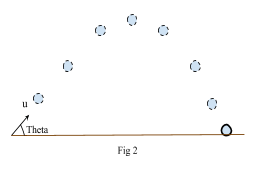# Projectiles

## Summary

• Projectile motion is basically the motion of an object that is moving in the air and experiences a gravitational force.
• The two types of projectile motions are; Horizontally launched projectiles & Non Horizontally launched projectiles.
• Important Kinematic Equations are:$v\quad \quad =\quad u\quad +\quad gt$${ v }^{ 2 }\quad =\quad { u }^{ 2 }\quad +\quad 2gs$$s\quad =\quad ut\quad +\quad \frac { 1 }{ 2 } g{ t }^{ 2 }$

Where v = final velocity, u = initial velocity, g = acceleration due to$gravity\quad =\quad 9.8\quad { m }/{ { s }^{ 2 } }$, t = total time and s=displacement.

### What is Projectile Motion?

Projectile motion is basically the motion of an object that is moving in the air and experiences a gravitational force. Examples of a few projectile motions are football, javelin and cannon balls as they all follow a parabolic trajectory which is a curved path and fall to the ground due to earth’s gravitational force.

To initiate a projectile motion, a force is applied to an object that causes the object to move and once moving on a curved path, the only two forces that act upon it are air resistance and gravitational force.

### There are two types of projectile motions:

• Horizontally launched projectiles: This type is when an object is launched horizontally from an elevated plane and follows a parabolic path to the ground as shown in Fig 1.• Non-horizontally launched projectiles: This is when an object is thrown in the air from the ground, rises to peak height and then drops to the ground again). In this case, at the maximum height, the vertical velocity vy is zero. The particle may still be moving horizontally but is not moving up or down.### Range

The range of a projectile motion is the total distance travelled horizontally.

The range of a projectile depends on its initial velocity denoted as u and launch angle theta ($\theta$ ).

Also note that range is maximum when$\theta$  = 45° as sin(2$\theta$) = sin (90) = 1.

Range can be calculated using the formula:$Range\quad =\quad \frac { { u }^{ 2 }sin(2\theta ) }{ g }$

### Time of Flight

The time of flight of a projectile motion is the time from when the object is projected to the time it takes for it to reach it to the surface. Time of velocity also depends on the initial velocity u and the angle of the projectile ‘theta’$\theta$ .

Consider the projectile motion in Fig 2 above. We say that the initial speed of the ball is defined by the vertical component of the speed defined as${ u }_{ y }\quad =\quad u\quad sin(\theta )$.

Using equation$s\quad =\quad ut\quad +\quad \frac { 1 }{ 2 } a{ t }^{ 2 }$  where s is the displacement which is 0 in this case, u is the initial speed${ u }_{ y }\quad =\quad u\quad sin(\theta )$,  and a =  gravitational acceleration (-g).$0\quad =\quad t(u\quad sin(\theta ))\quad -\quad \frac { 1 }{ 2 } g{ t }^{ 2 }$$0\quad =\quad t\left[ u\quad sin(\theta )\quad -\quad \frac { 1 }{ 2 } gt \right]$

Hence:

t = 0 or$t\quad =\quad \frac { 2u\quad sin(\theta ) }{ g }$$Time\quad of\quad Flight\quad =\quad \frac { 2u\quad sin(\theta ) }{ g }$

#### Example #1

Q. A cannonball is fired straight into the air with an initial velocity of 20 m/s.i) What is the maximum height the cannonball reaches.
ii) How long does it take for the cannonball to return to its initial position.

Solution:

i) We know that when the ball reaches its maximum height it stops for a fraction of a second before starting to come back to its initial position. Therefore we can say that the final velocity when the cannonball reaches its maximum height is zero.

v = 0 m/s

From the question we know the following data:

Initial velocity u = 20 m/s and acceleration due to gravity is -9.8${ m }/{ { s }^{ 2 } }$, negative sign is there because the ball is projected upwards.

To find the maximum height we use the equation${ v }^{ 2 }\quad =\quad { u }^{ 2 }\quad +\quad 2as$  where s is the distance travelled which is also the maximum height.

We can now substitute the values:${ 0 }^{ 2 }\quad =\quad { 20 }^{ 2 }\quad +\quad 2(9.8)s$

400 = 19.6 s

s = 20.4 m

Hence:

Maximum height = 20.4 m       Ans

ii) In this part we have to find the total time of flight which is also known as the total time the cannonball was in the air. We know that the time it takes the object to go up is equal to the time it will take for it to come down to its initial position.

Hence, we first calculate the time it took the cannonball to reach its maximum height and then we multiply it by 2 to get the total time of flight.

We can see that the most suitable kinematic equation to apply here is:

v = u + at

As we know v = 0 m/s, u = 20 m/s, and a = g = -9.8${ m }/{ { s }^{ 2 } }$

0 = 20 – 9.8 t${ t }_{ up }\quad =\quad 2.04\quad seconds$$Total\quad time\quad of\quad Flight{ \quad =\quad 2t }_{ up }$

= 2(2.04)

= 4.08 seconds           Ans